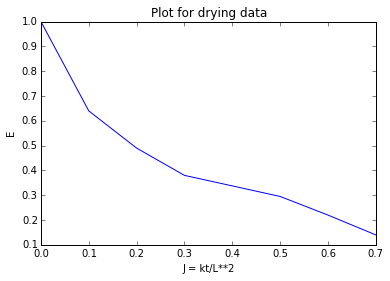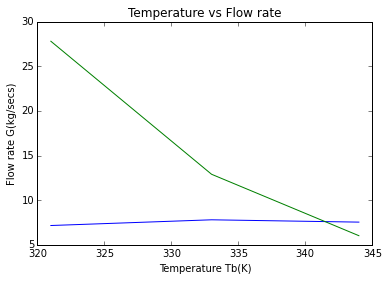# Chapter 16 - Drying¶

## Page 909 Example 16.1¶

In :
from math import log
from __future__ import division
#For the first drying operation
w1 = 0.25#                 #in kg/kg
w = 0.10#                  #in kg/kg
wc = 0.15#                 #in kg/kg
we = 0.05#                 #in kg/kg
f1 = w1-we#                #in kg/kg
fc = wc - we#              #in kg/kg
f = w - we#                #in kg/kg

#x = mA
def TotalDryingTime(t):
x = (1/t)*((f1-fc)/fc + log(fc/f))
return x

print"\n     mA = %.3f kg/sec"%(TotalDryingTime(15))

#For the second drying operation
w12 = 0.30#                  #in kg/kg
w2 = 0.08#                   #in kg/kg
wc2 = 0.15#                  #in kg/kg
we2 = 0.05#                  #in kg/kg
f12 = w12 - we2#             #in kg/kg
fc2 = wc2 - we2#             #in kg/kg
f2 = w2 - we2#               #in kg/kg

t1=(1/TotalDryingTime(15))*((0.25-0.10)/0.10 + log(0.10/0.03))
print"\n The total drying time is then %.1f ksec or %.2f hr "%(t1,t1*1000/3600)

     mA = 0.113 kg/sec

The total drying time is then 24.0 ksec or 6.65 hr


## Page 909 Example 16.2¶

In :
%matplotlib inline
from matplotlib.pyplot import plot,title,show,xlabel,ylabel
from sympy import symbols,solve

E = [1, 0.64 ,0.49, 0.38, 0.295, 0.22, 0.14]
J = [0, 0.1, 0.2, 0.3, 0.5, 0.6 ,0.7]
plot(J,E)
title("Plot for drying data")
xlabel("J = kt/L**2")
ylabel("E")
show()

#For the 10 mm strips
mi = (0.28 - 0.07)#        #Initial free moisture content in kg/kg
mf = (0.13-0.07)#          #Final free moisture content in kg/kg
#at
t = 25#                    #time is in ksecs
E = (0.06/0.21)
#at E = 0.286 ,J = 0.52 from the plot of given data and J = kt/L**2
k = symbols('k')
k1 = solve(0.52 - (k*t)/(10/2)**2)
print"\n k = %f"%(k1)

#for the 60 mm planks
m1i = (0.22 - 0.07)#       #Initial free moisture content in kg/kg
m1f = (0.13 - 0.07)#       #Final free moisture content in kg/kg
E = (m1f/m1i)
#at E = 0.20 from yhe plot of the given data J = 0.63 and J = kt/L**2
t1 = 0.63*(60/2)**(2)/k1
print"\n t1 = %d ksecs or %.1f days"%(t1,(t1*1000/(3600*24)))k = 0.520000

t1 = 1090 ksecs or 12.6 days


## Page 911 Example 16.3¶

In :
from sympy import symbols,solve

#(a) Air
#G kg/s dry air enter with 0.006G kg/s water vapour and hence the heat content of this stream=
#[(1.00G) + (0.006G × 2.01)](385 − 273) = 113.35G kW

#(b) Wet solid
#0.125 kg/s enter containing 0.40 kg water/kg wet solid, assuming the moisture is expressed on a wet basis.
flowWater = (0.125*0.40)#               #in kg/sec
flowDrySolid = (0.125-0.050)#           #in kg/sec
#Hence heat content of this stream
q = ((0.050*4.18)+(0.075*0.88))*(295-273)
print"\n The heat content of this stream = %.2f kW"%(q)

#Heat out
#(a) Air
#Heat in exit air = [(1.00 G) + (0.006 G × 2.01)](310 − 273) = 37.45G kW.
fd = 0.075#               #mass flow rate of dry solids in kg/sec
w = 0.05*0.075/(1+0.05)#  #water in the dried solids leaving in kg/secs
we = (0.050 - w)#         #The water evaporated into gas stream in kg/secs

#Assuming evaporation takes place at 295 K,then:
qout = 0.0464*(2.01*(310-295)+2449+4.18*(295-273))
print"\n Heat in the water vapour = %.1f kW"%(qout)

#the total heat in this stream = (119.30 + 37.45G) kW.
#(b) Dried solids

#The dried solids contain 0.0036 kg/s water and hence heat conten     t of this stream is:
q1 = ((0.075*0.88)+(0.0036*4.18)/(305-273))
print"\n The dried solids contain 0.0036 kg/s water and hence heat content of this stream is: %.2f kW"%(q1)

#(c) Losses
#These amount to 20 kJ/kg dry air or 20m kW.
#Heat Balance
G = symbols('G')
G1 = solve(113.35*G + 6.05 - 119.30 - 37.45*G - 2.59 - 20*G)
print"\n G = %.2f kg/secs"%(G1)
print"\n Water in the outlet stream %.4f kg/secs"%(0.006*2.07+0.0464)
print"\n The humidity H = %.4f kg/kg dry air"%(0.0588/2.07)

 The heat content of this stream = 6.05 kW

Heat in the water vapour = 119.3 kW

The dried solids contain 0.0036 kg/s water and hence heat content of this stream is: 0.07 kW

G = 2.07 kg/secs

Water in the outlet stream 0.0588 kg/secs

The humidity H = 0.0284 kg/kg dry air


## Page 922 Example 16.4¶

In :
from sympy import symbols,solve

#In 100 kg of feed

#mass of water =
mw = 100*30/100#                  #mass of water in kg
#mass of dry solids =
md = 100-30#                      #mass of dry solids

#and:
#For b kg water in the dried solids: 100b/(b + 70) = 15.5
b = symbols('b')
b1 = solve(100*b - 15.5*(b+70))
print"\n water in the product ,b = %.1f kg"%(b1)

#Initial water content
w1 = 30/70#            #Intial moisture content in kg/kg dry solids
#Final moisture content
w2 = (12.8/70)#        #Final moisture content in kg/kg dry solids
#water to be removed
w3 = (30-12.8)#        #water to be removed in kg

#Surface of drying
S = (0.03*70)#            #Surface for drying in m**2
rate = (0.0007*2.1)#      #Rate of drying in kg/sec

#As the final moisture content is above the critical value, all the drying is at this constant rate and the time of drying is:
t = 17.2/0.00147
print"\n As the final moisture content is above the critical value, all the drying is at this constant rate and the time of drying is: %d ksecs or %.2f hr"%(t,t/3600)

 water in the product ,b = 12.8 kg

As the final moisture content is above the critical value, all the drying is at this constant rate and the time of drying is: 11700 ksecs or 3.25 hr


## Page 930 Example 16.5¶

In :
from sympy import symbols,solve
from math import exp,log,pi

#Inlet air temperature
Tair = 400#            #Inlet air temperature in kelvins
H = 0.01#              #Humidity is in kg/kg dry air
#*therefore wet bulb temperature =
Twetbulb = 312#        #Inlet wet-bulb temperature
NTU = 1.5#              #Number of transfer units

#Then for adiabatic drying the outlet air temperature,To is given by
To = symbols('To')
To1 = solve(exp(1.5)*(To-312)-(400-312))
print"\n For adiabatic drying the outlet air temperature = %.1f K"%(To1)

#Solids outlet temperature will be taken to be maximum allowable,325K
#From the steam tables in the Appendix, the latent heat of vaporisation of water at 312 K is2410 kJ/kg. Again from steam tables, the specific heat capacity of water vapour = 1.88 kJ/kg K and that of the solids will be taken as 2.18 kJ/kg K.

#For a mass flow of solids of 0.35 kg/s and inlet and outlet moisture contents of 0.15 and 0.005 kg/kg dry solids respectively, the mass of water evaporated = 0.35(0.15 − 0.005) = 0.0508 kg/s.

#For unit mass of solids ,the heat duty includes:
#Heat to the solids
qsolids = 2.18*(325-300)#       #heat to solids in kJ/kg
#Heat to raise the moisture to the dew point
qdew = (0.15*4.187*(312-300))#  #in kJ/kg
#Heat of vaporisation
qvap = 2410*(0.15-0.005)#       #in kJ/kg
#Heat to raise remaining moisture to solids outlet temperature
qremaining = (0.05*4.187)*(325-312)
#Heat to raise evaporated moisture to the air outlet temp.
qevapo = (0.15-0.005)*1.88*(331.5-312)
qtotal = qsolids + qdew + qvap + qremaining + qevapo
print"\n Total heat = %.1f kJ/kg or %d kW"%(qtotal,qtotal*0.35)

#The humid heat of entering air is 1.03 kJ/kg K
#G1 (1 +H1) = Q/Cp1(T1 − T2)
#where: G1 (kg/s) is the mass flowrate of inlet air,
#H1 (kg/kg) is the humidity of inlet air,
#Q (kW) is the heat duty,
#Cp1 (kJ/kg K) is the humid heat of inlet air
#and: T1 and T2 (K) are the inlet and outlet air temperatures         respectively.
G1 = symbols('G1')
G = solve(G1*(1+0.01)-146/(1.03*(400-331.5)))
print"\n Mass flow rate of inlet air = %.2f kg/secs"%(G)
print"\n Mass flow rate of dry air ,Ga = %.2f kg/secs"%(G/1.01)
print"\n the humidity of the outlet air H2 = %.4f kg/kg"%(0.01+0.0508/2.05)

#At a dry bulb temperature of 331.5 K, with a humidity of 0.0347 kg/kg, the wet-bulb temperatureof the outlet air, from Figure 13.4 in Volume 1, is 312 K, the same as the inlet, which is the case for adiabatic drying.

#The dryer diameter is then found from the allowable mass velocity of the air and the entering air flow and for a mass velocity of 0.95 kg/m**2.secs, the cross sectional area of the dryer is
print"\n The cross sectional area of the dryer is %.2f m**2"%(2.07/0.95)
print"\n The equivalent diameter of the dryer = %.2f m"%(((4*2.18)/pi)**0.5)

#With a constant drying temperature of 312 K:
#at the inlet
deltaT1 = 400-312#           #inlet temperature is in deg K
#at the outlet
deltaT2 = 331.5-312#         #outlet temperature is in deg K
Tlogmean = (deltaT1 - deltaT2)/log(deltaT1/deltaT2)
print"\n Logarithmic mean temperature difference = %.1f deg K"%(Tlogmean)
#The length of the dryer, L is then: L = Q/(0.0625πDG**(0.67)*Tm)
#where D (m) is the diameter
#and G(kg/m**2.secs) is the air mass velocity.
L = 146/(0.0625*(pi)*1.67*(0.95)**(0.67)*45.5)
print"\n The length of the dryer = %.1f m"%(L)
print"\n Length/diameter ratio = %d "%(10.1/1.67)

 For adiabatic drying the outlet air temperature = 331.6 K

Total heat = 419.5 kJ/kg or 146 kW

Mass flow rate of inlet air = 2.05 kg/secs

Mass flow rate of dry air ,Ga = 2.03 kg/secs

the humidity of the outlet air H2 = 0.0348 kg/kg

The cross sectional area of the dryer is 2.18 m**2

The equivalent diameter of the dryer = 1.67 m

Logarithmic mean temperature difference = 45.5 deg K

The length of the dryer = 10.1 m

Length/diameter ratio = 6


## Page 950 Example 16.6¶

In :
from math import sqrt
%matplotlib inline
from matplotlib.pyplot import plot,title,show,xlabel,ylabel

H = 0.036#               #Humidity is in kg/kg at 811 K
#Taking R as 90 per cent and P as 101.3 kN/m2, then, for assumed values of Tb of 321, 333 and 344 K
#Pw = 13,20 and 32 kN/m2, respectively
#G = 27.8, 12.9 and 6.02 kg/s, respectively.

#for Tb = 321, 333 and 344 K,
#G = 7.16, 7.8 and 7.54 kg/s respectively.
Tb = [321, 333, 344]
G1 = [27.8 ,12.9, 6.02]#           #Temperature is in kelvins
G = [7.16 ,7.8, 7.54]#             #flow rate in kg/secs
plot(Tb,G)
plot(Tb,G1)
title("Temperature vs Flow rate")
xlabel("Temperature Tb(K)")
ylabel("Flow rate G(kg/secs)")
show()

#Plotting G against Tb for each equation on the same axis, then
Go = 8.3#                         #Gas flow rate is in kg/secs
Tb = 340#                         #temperature is in Kelvins
uf = 0.61#                        #velocity is in m/secs

D = sqrt(340*(8.3+(1.58*1.26))/(278*0.61))
print"\n D = %.2f m"%(D)D = 4.54 m